# Fluid Continuity

According to the equations of marginally-stable ideal-MHD, the electron number density continuity equation takes the form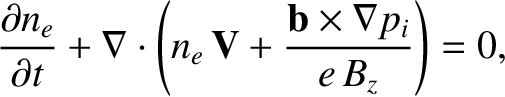(3.45)

where use has been made of Equations (2.139), (2.380), and (3.26). Here,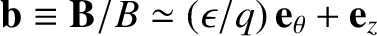. It follows that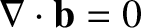and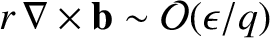. Thus,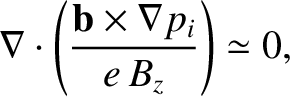(3.46)

where use has been made of Equation (3.29).

Now, the equilibrium plasma flow is written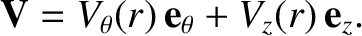(3.47)

It follows that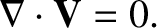(3.48)

The linearized form of Equation (3.45) is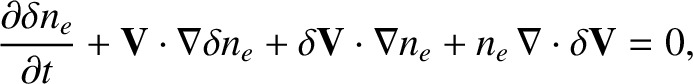(3.49)

where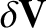is the perturbed plasma velocity, and use has been made of Equations (3.46) and (3.48). Let us write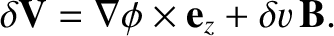(3.50)

It follows that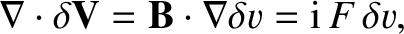(3.51)

where use has been made of Equations (3.8) and (3.19). Hence, writing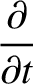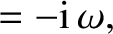(3.52)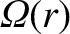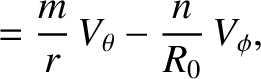(3.53)

Equation (3.49) reduces to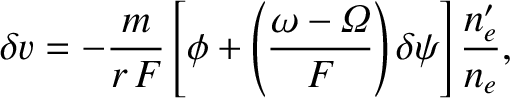(3.54)

where use has been made of Equation (3.42).